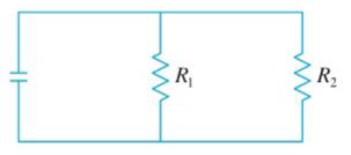Chapter 3.9, Problem 39E

Chapter
Section
Textbook Problem

If two resistors with resistances R1 and R2 are connected in parallel, as in the figure, then the total resistance R, measured in ohms (Ω), is given by 1 R = 1 R 1 + 1 R 2 If R1 and R2 arc increasing at rates of 0.3 0Ω/s and 0.2 Ω/s, respectively, how fast is R changing when R1 = 80 Ω and R2 = 100Ω?To determine

To find: The rate of change of the total resistance R of the circuit.

Explanation

Given:

The rate of change of the resistance R1 is 0.3Ω/s .

The rate of change of the resistance R2 is 0.2Ω/s .

Formula used:

(1) Chain rule: dydx=dydududx.

(2) ddx[fg]=gdfdxfdgdx[g]2.

Calculation:

Since the resistance R1 and R2 are connected in the parallel combination and R is the total equivalent resistance of the given circuit.

Therefore,

1R=1R1+1R2 .

Now first find R.

1R=180+11001R=5+44001R=9400R=4009Ω

Consider the resistance R1 and R2 changes with time t, therefore the total resistance R is also changes with the time t.

Since dR1dt=0.3Ω/s and dR2dt=0.2Ω/s .

Find dRdt when R1=80Ω and R2=100Ω

Differentiate 1R=1R1+1R2 with respect to the time t.

ddt[1R]=ddt[1R1+1R2]ddR[1R]dRdt=ddR1[1R1]dR1dt+ddR2[1R2]dR2dt[Qdydx=dydududx]1R2dRdt=1R12dR1dt+(1R22)dR2dt

Still sussing out bartleby?

Check out a sample textbook solution.

See a sample solution

The Solution to Your Study Problems

Bartleby provides explanations to thousands of textbook problems written by our experts, many with advanced degrees!

Get Started

Expand each expression in Exercises 122. (2xy)y

Finite Mathematics and Applied Calculus (MindTap Course List)

In Exercises 6372, evaluate the expression. 63. |6 + 2|

Applied Calculus for the Managerial, Life, and Social Sciences: A Brief Approach

In problems 23-58, perform the indicated operations and simplify. 40.

Mathematical Applications for the Management, Life, and Social Sciences

True or False: is a convergent series.

Study Guide for Stewart's Multivariable Calculus, 8th